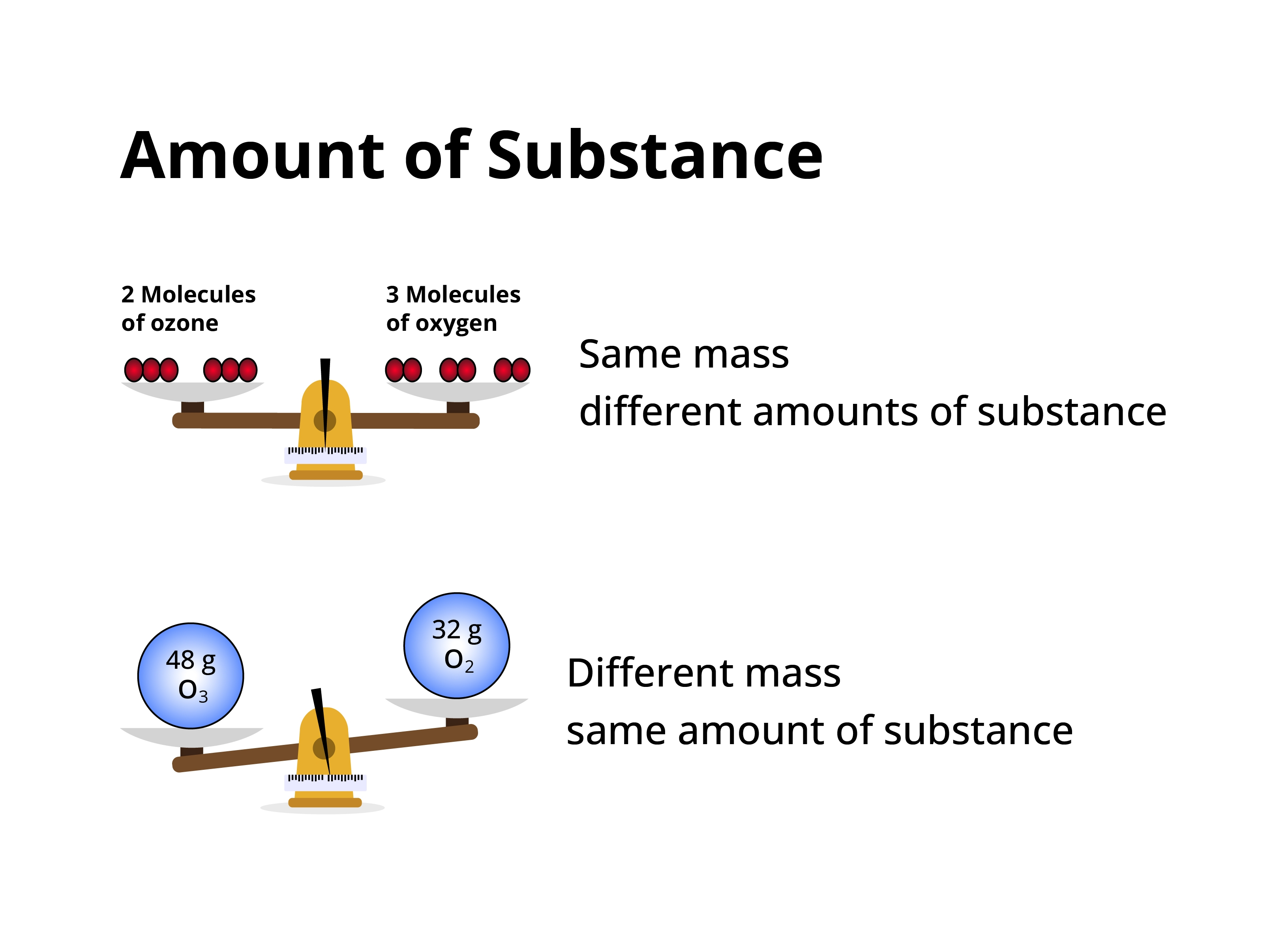# Amount of Substance Calculator

Input the value of Mass (g) and Molar mass (g/mol) to find the amount of substance (Mass/Molar Mass) using this amount of substance calculator.

Formula:
Amount of Substance = Mass (m)Molar mass (M)

g
g/mol

Give Us Feedback

## How to use this Amount of Substance Calculator?

To calculate the amount of substance in a mole follow the below steps:

• Input the value of Mass (g) and molar mass (g/mol).
• Press the calculate button.